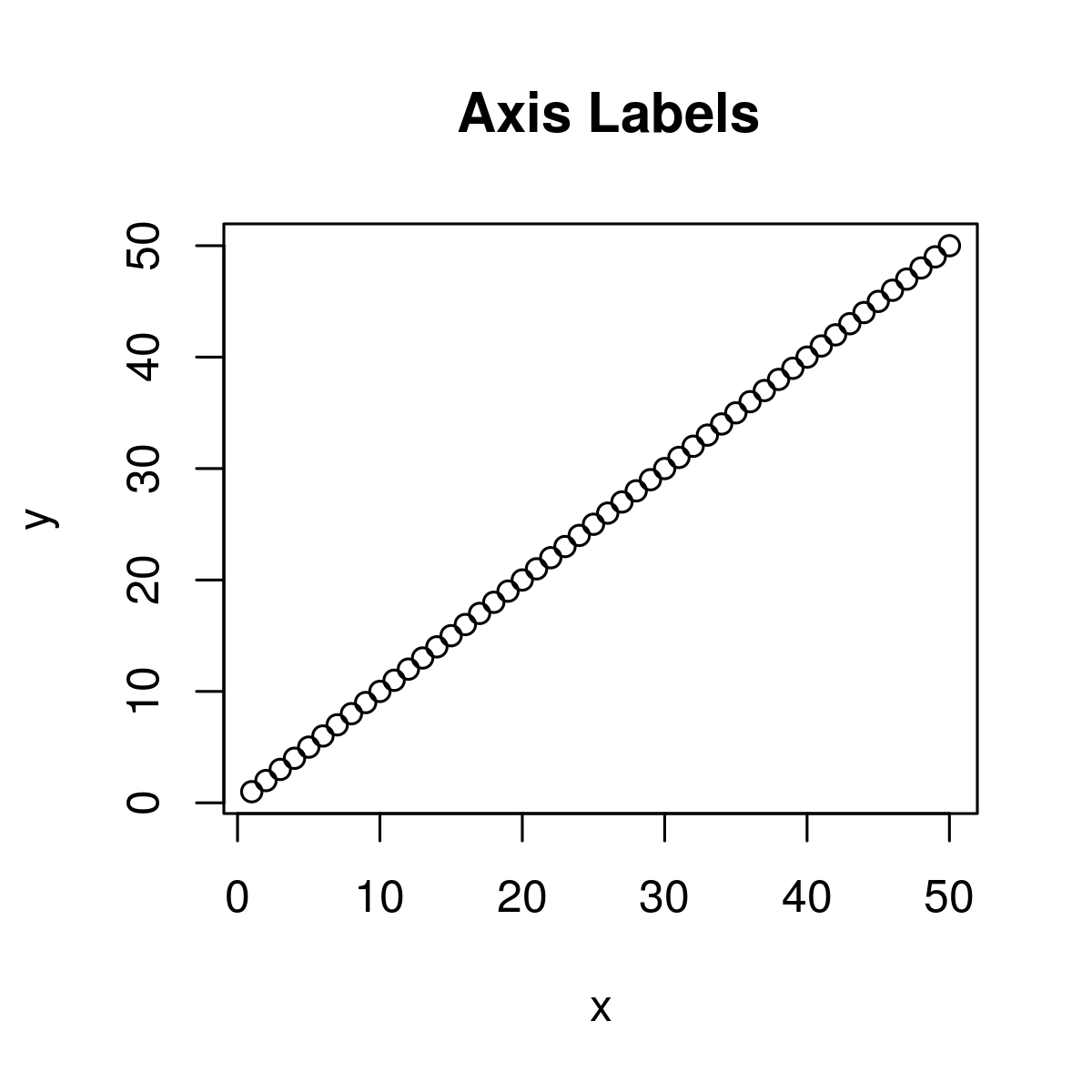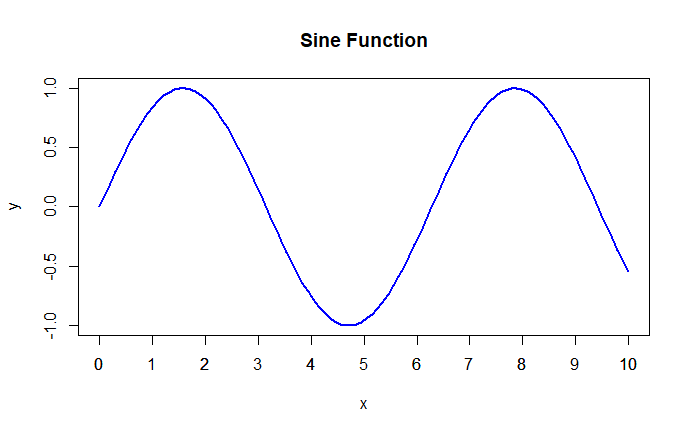GFG App
Open AppBrowser
Continue

# Compute a Sequence of Equally Spaced Round values in R Programming – pretty() Function

pretty() function in R Language is used to decide sequence of equally spaced round values.

Syntax: pretty(x, n) Parameters: x: It is defined as vector data n: length of resultant vector Returns: data vector of equal length interval

Example 1:

## r

 `# Create example vector` `# Apply pretty function to vector` `x <- 1:50                                 ` `pretty``(x)                                     `

Output:

`  0 10 20 30 40 50`

Example 2:

## r

 `# Create example vector` `x <- 1:50     `   `# Apply pretty function to vector                            ` `pretty``(x, n = 10)                   `

Output:

```
  0  5 10 15 20 25 30 35 40 45 50```

Here n = 10 states that the interval vector consisting of ten intervals, i.e. n+1 elements. Example 3:

## r

 `# Create example vectors` `x <- 1:50 ` `y <- 1:50   `   `# Create plot of two vectors                       ` `plot``(x, y,        ` `   `  `     ``# Default axis labels                     ` `     ``main = " Axis Labels")             `

Output:Example :

## R

 `# Generate some data` `x <- ``seq``(0, 10, length.out = 100)` `y <- ``sin``(x)`   `# Create a plot of the data` `plot``(x, y, type = ``"l"``, col = ``"blue"``, lwd = 2, main = ``"Sine Function"``,` `     ``xlab = ``"x"``, ylab = ``"y"``)`   `# Add tick marks to the x-axis` `ticks <- ``pretty``(x, n = 10)` `axis``(1, at = ticks, labels = ``round``(ticks, 2))`

output :My Personal Notes arrow_drop_up